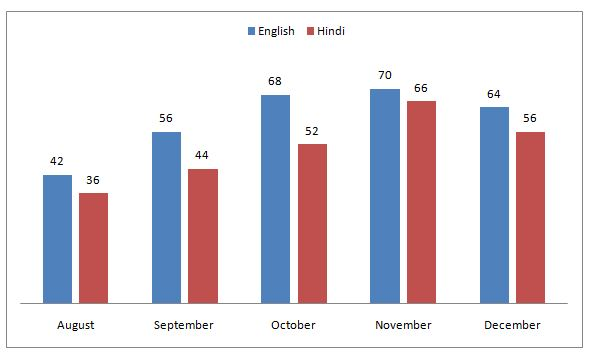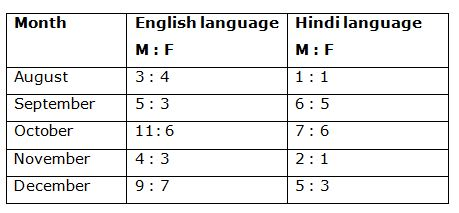# IBPS PO Mains 2018 – Quantitative Aptitude Questions Day-25

Dear Readers, Bank Exam Race for the Year 2018 is already started, To enrich your preparation here we have providing new series of Practice Questions on Quantitative Aptitude – Section. Candidates those who are preparing for IBPS PO Mains 2018 Exams can practice these questions daily and make your preparation effective.

[WpProQuiz 4261]

Directions (Q. 1 – 5): The following questions are accompanied by three statements. You have to determine which statement is/are sufficient to answer the questions.

1) In how many days A and B can together complete the work?

I. A is twice as efficient as B.

II. A can complete the work alone in 24 days.

III. A and B together complete 3/4 of the work in 12 days.

a) If statement I and II are sufficient

b) If III and either I or II are sufficient

c) Either I and II together or III alone is sufficient

d) If any two of I, II and III are sufficient

e) If all statements together are sufficient

2) What is the age of the class teacher?

I. The average age of 14 students in the class is 18 years.

II. The average increases by 2 when the age of the class teacher is included.

III. The age of the teacher is 20 years more than the eldest boy in the class.

a) If statements I and II together are sufficient

b) If III and either I or II are sufficient

c) Either I and II together or III alone is sufficient

d) If any two of I, II and III are sufficient

e) If all statements together are sufficient

3) What is the length of the platform?

I. The train crosses an electric pole in 12 seconds.

II. The train crosses a platform in 20 seconds

III. The speed of the train is 30m/s.

a) If statement I and II are sufficient

b) If III and either I or II are sufficient

c) Either I and II together or III alone is sufficient

d) If any two of I, II and III are sufficient

e) If all statements together are sufficient

4) What are the minimum marks is required to pass the examination?

I. Anitha scored 30% marks in the examination and failed by 20 marks while his friend Avinash scored 40% marks and got 15 marks more than the passing marks.

II. Prema scored 40% marks in the examination.

III. A student has to score minimum 35.71% marks to pass the examination.

a) If only statement I is sufficient

b) If either I or III alone are sufficient

c) If statement II and III together is sufficient

d) If statement I and III together is sufficient

e) All the statements are required.

5) What is the rate of interest?

I. The difference between the compound interest and simple interest on an amount of Rs. 25000 in 2 years is Rs. 360.

II. An amount doubles itself at simple rate of interest in 8(1/3) years.

III. The compound interest accrued in 10 years is more than the principal.

a) If only statement I is sufficient

b) If either I or II alone are sufficient

c) If statement II and III together is sufficient

d) If statement I and III together is sufficient

e) All the statements are required

Directions (Q. 6 – 10) Study the following information carefully and answer the given questions:

The following bar graph shows the total number of newspaper readers (In thousands) of English and Hindi language in different months of a certain city in the year 2017 and the table shows the ratio of male to female readers among them.6) Total number of male Hindi newspaper readers in the month of August and November together is approximately what percentage of total number of female English newspaper readers in the month of September and December together?

a) 113 %

b) 95 %

c) 127 %

d) 81 %

e) 133 %

7) Find the ratio between the total number of male Hindi newspaper readers to that of total number of female English newspaper readers of all the given months together?

a) 155 : 124

b) 149 : 127

c) 121 : 132

d) 117 : 98

e) None of these

8) Find the average number of female Hindi newspaper readers of all the given months together?

a) 21000

b) 27000

c) 18000

d) 32000

e) None of these

9) Find the difference between the total English newspaper readers to that of total Hindi newspaper readers of all the given months together?

a) 38000

b) 34000

c) 46000

d) 42000

e) None of these

10) Total number of female Hindi newspaper readers in the month of September and October together is approximately what percentage more/less than the total number of male English newspaper reader in the month of November and December together?

a) 54 % more

b) 42 % less

c) 34 % more

d) 34 % less

e) 42 % more

From I and II,

A= 24 days

B = 48 days

A and B’s one day work = 1/24 + 1/48 = 1/16

A and B together can complete the work in 16 days.

From III,

¾ of work = 12

Whole work of A and B = 12*(4/3) = 16 days

From I and II,

Total ages of students without class teacher = 14*18= 252 years

Total ages including class teacher = 20*15 = 300 years

The age of the class teacher = 300 – 252 = 48 years

Let the length of the platform be x,

From I and III,

Train length/12 = 30

Length of the train =12*30= 360 m

From II,

20 = (360 + x)/30

600= 360 + x

X = 240m

All the statements together are sufficient

From statement I,

Let the maximum marks in the examination be x,

According to the question,

30 % of x + 20 = 40% of x – 15

30x/100 + 20 = 40x/100 – 15

35 = 10x/100

X = 35*(100/10)

X = 350

Minimum marks required to pass the examination

= > 30*(350/100) + 20 = 125

Only statement I is alone sufficient.

From statement I,

Diff = Sum*(r/100)2

r2 = (360*10000)/25000

r2 = 144

Rate of interest = 12%

From statement II,

Let the principal be x,

Amount = 2x

S.I = 2x – x = x

Time= 25/3 years

Rate of interest = (x*100*3)/(25*x)

R = 12 %

Total number of male Hindi newspaper readers in the month of August and November together

= > 36000*(1/2) + 66000*(2/3)

= > 18000 + 44000 = 62000

Total number of female English newspaper readers in the month of September and December together

= > 56000*(3/8) + 64000*(7/16)

= > 21000 + 28000 = 49000

Required % = (62000/49000)*100 = 126.53 % = 127 %

The total number of male Hindi newspaper readers of all the given months together

= > [36*(1/2) + 44*(6/11) + 52*(7/13) + 66*(2/3) + 56*(5/8)] thousand

= > [18 + 24 + 28 + 44 + 35] thousand

= > 149000

The total number of female English newspaper readers of all the given months together

= > [42*(4/7) + 56*(3/8) + 68*(6/17) + 70*(3/7) + 64*(7/16)] thousand

= > [24 + 21 + 24 + 30 + 28] thousand

= > 127000

Required ratio = 149000 : 127000 = 149 : 127

The total number of female Hindi newspaper readers of all the given months together

= > [36*(1/2) + 44*(5/11) + 52*(6/13) + 66*(1/3) + 56*(3/8)] thousand

= > [18 + 20 + 24 + 22 + 21] thousand

= > 105000

Required average = 105000/5 = 21000

The total English newspaper readers of all the given months

= > [42 + 56+ 68 + 70 + 64] thousand

= > 300000

The total Hindi newspaper readers of all the given months

= > [36 + 44 + 52 + 66 + 56] thousand

= > 254000

Required difference = 300000 – 254000 = 46000

Total number of female Hindi newspaper readers in the month of September and October together

= > [44*(5/11) + 52*(6/13)] thousand

= > [20 + 24] thousand

= > 44000

Total number of male English newspaper reader in the month of November and December together

= > [70*(4/7) + 64*(9/16)] thousand

= > [40 + 36] thousand = 76000

Required % = [(76000 – 44000)/76000]*100 = 42.1 % = 42 % less

Daily Practice Test Schedule | Good Luck

 Topic Daily Publishing Time Daily News Papers & Editorials 8.00 AM Current Affairs Quiz 9.00 AM Current Affairs Quiz (Hindi) 9.30 AM IBPS Clerk Prelims – Reasoning 10.00 AM IBPS Clerk Prelims – Reasoning (Hindi) 10.30 AM IBPS Clerk Prelims – Quantitative Aptitude 11.00 AM IBPS Clerk Prelims – Quantitative Aptitude (Hindi) 11.30 AM Vocabulary (Based on The Hindu) 12.00 PM IBPS Clerk Prelims – English Language 1.00 PM SSC Practice Questions (Reasoning/Quantitative aptitude) 2.00 PM IBPS PO/Clerk – GK Questions 3.00 PM SSC Practice Questions (English/General Knowledge) 4.00 PM Daily Current Affairs Updates 5.00 PM IBPS PO Mains – Reasoning 6.00 PM IBPS PO Mains – Quantitative Aptitude 7.00 PM IBPS PO Mains – English Language 8.00 PM